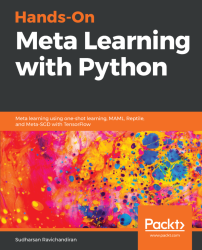•#### Hands-On Meta Learning with Python#### Overview of this book

Meta learning is an exciting research trend in machine learning, which enables a model to understand the learning process. Unlike other ML paradigms, with meta learning you can learn from small datasets faster. Hands-On Meta Learning with Python starts by explaining the fundamentals of meta learning and helps you understand the concept of learning to learn. You will delve into various one-shot learning algorithms, like siamese, prototypical, relation and memory-augmented networks by implementing them in TensorFlow and Keras. As you make your way through the book, you will dive into state-of-the-art meta learning algorithms such as MAML, Reptile, and CAML. You will then explore how to learn quickly with Meta-SGD and discover how you can perform unsupervised learning using meta learning with CACTUs. In the concluding chapters, you will work through recent trends in meta learning such as adversarial meta learning, task agnostic meta learning, and meta imitation learning. By the end of this book, you will be familiar with state-of-the-art meta learning algorithms and able to enable human-like cognition for your machine learning models.
Title PageDedicationContributorsPrefaceFree Chapter
Introduction to Meta LearningFace and Audio Recognition Using Siamese NetworksPrototypical Networks and Their VariantsRelation and Matching Networks Using TensorFlowMemory-Augmented Neural NetworksMAML and Its VariantsMeta-SGD and ReptileGradient Agreement as an Optimization ObjectiveAssessmentsOther Books You May EnjoyIndex## Gradient agreement as an optimization

The gradient agreement algorithm is an interesting and recently introduced algorithm that acts as an enhancement to meta learning algorithms. In MAML and Reptile, we try to find a better model parameter that's generalizable across several related tasks so that we can learn quickly with fewer data points. If we recollect what we've learned in the previous chapters, we've seen that we randomly initialize the model parameter and then we sample a random batch of tasks,. For each of the sampled tasks,, we minimize the loss by calculating gradients and we get the updated parameters,, and that forms our inner loop:After calculating the optimal parameter for each of the sampled tasks, we perform meta optimization— that is, we perform meta optimization by calculating loss in a new set of tasks, we minimize loss by calculating gradients with respect to the optimal parameters, which we obtained in the inner loop, and we update our...